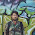## Tuesday, June 24, 2014

### GLSL Classes

GLSL is not object oriented, there are no classes or method calls. GLSL4.0 has subroutine variables that can provide an object oriented solution, but this is not compatible with GLSL in WebGL.

### PythonJS GPU Class

In the example below an array of MyObject is created and uploaded to the GPU, where it is iterated over. The method call s.mymethod(1.1, 2.2) translates to MyObject_mymethod(s, 1.1, 2.2) in the GLSL shader.

```@gpu.object
class MyObject:
@gpu.method
float def subroutine(self, x,y):
float x
float y
return x + y * self.attr2

@gpu.method
float def mymethod(self, x,y):
float x
float y
if self.index == 0:
return -20.5
elif self.index == 0:
return 0.6
else:
return self.subroutine(x,y) * self.attr1

def __init__(self, a, b, i):
self.attr1 = a
self.attr2 = b
self.index = int16(i)

class myclass:
def run(self, w):
self.array = [MyObject(1.1,1.2,x) for x in range(w)]

@returns( array=64 )
@gpu.main
def gpufunc():
struct* A = self.array
float b = 0.0

for s in iter(A):
b += s.mymethod(1.1, 2.2)

return b

return gpufunc()
```

1.1.